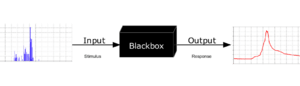# Mathematical model facts for kids

Kids Encyclopedia FactsTo analyse something with a typical "black box approach", only the behavior of the stimulus/response will be accounted for, to infer the (unknown) box. The usual representation of this black box system is a data flow diagram centered in the box.

A mathematical model is a description of a system using mathematical concepts and language. The process of building a mathematical model is termed mathematical modeling. Mathematical models are used in the natural sciences (such as physics, biology, earth science, meteorology) and engineering disciplines (e.g. computer science, artificial intelligence). They are also used in the social sciences (such as economics, psychology, sociology and political science). Physicists, engineers, statisticians, operations research analysts and economists use mathematical models a lot.

Mathematical models can take many forms. The types of models include:

• dynamical systems - for systems that change,
• statistical models - for finding patterns in large groups of measurements or data,
• differential equations - to study how variables change over time, or
• game theoretic models - for studying how many independent decision makers can interact.

These and other types of models can overlap, with a given model involving a variety of abstract structures. Mathematical models can include logical models. In many cases, the quality of a scientific field depends on how well the mathematical models built on theory agree with results of repeatable experiments. When theoretical mathematical models do not match experimental measurements, scientists try to correct the model. Such corrections lead the way to better theories to explain the facts.Mathematical model Facts for Kids. Kiddle Encyclopedia.